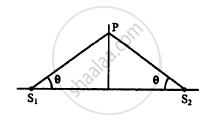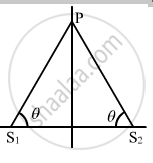Department of Pre-University Education, KarnatakaPUC Karnataka Science Class 11
Advertisement Remove all ads

# Two Sources of Sound S1 and S2 Vibrate at Same Frequency and Are in Phase. the Intensity of Sound Detected at a Point P as Shown in the Figure is I0. - Physics

Sum

Two sources of sound S1 and S2 vibrate at same frequency and are in phase. The intensity of sound detected at a point P as shown in the figure is I0. (a) If θ equals 45°, what will be the intensity of sound detected at this point if one of the sources is switched off? (b) What will be the answer of the previous part if θ = 60°?Advertisement Remove all ads

#### Solution

Given:
Resultant intensity at P = I0
The two sources of sound S1 and S2 vibrate with the same frequency and are in the same phase.
(a) When θ = 45°:
Path difference = S1P − S2P = 0So, when the source is switched off, the intensity of sound at P is $\frac{I_0}{4}$ .

(b) When θ = 60°, the path difference is also 0. Similarly, it can be proved that the intensity at P is $\frac{I_0}{4}$

when the source is switched off.

Concept: Wave Motion
Is there an error in this question or solution?
Advertisement Remove all ads

#### APPEARS IN

HC Verma Class 11, 12 Concepts of Physics 1
Chapter 16 Sound Waves
Q 35 | Page 355
Advertisement Remove all ads
Advertisement Remove all ads
Share
Notifications

View all notifications

Forgot password?